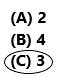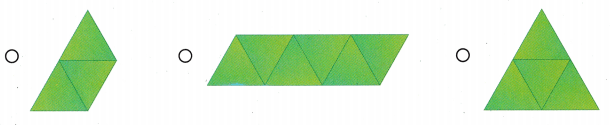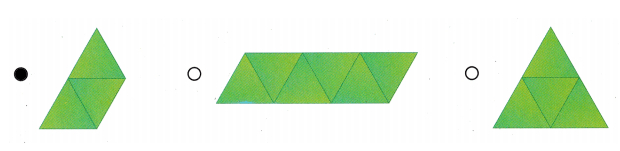# Texas Go Math Grade 1 Lesson 14.3 Answer Key Create Two-Dimensional Shapes

Refer to our Texas Go Math Grade 1 Answer Key Pdf to score good marks in the exams. Test yourself by practicing the problems from Texas Go Math Grade 1 Lesson 14.3 Answer Key Create Two-Dimensional Shapes.

## Texas Go Math Grade 1 Lesson 14.3 Answer Key Create Two-Dimensional Shapes

Explore

Use pattern blocks. Draw to show your work.
Answer: A set of pattern blocks typically consists of six different shapes in six different colours. This includes a yellow hexagon, red trapezoid, green equilateral triangle, blue rhombus, orange square, and thin beige rhombus. Except for the trapezoid, the lengths of all sides of each shape are the same.FOR THE TEACHER • Have children use pattern blocks to act out the following problem. Karen has some green pattern blocks. She made a shape with 3 sides and a shape with 4 sides. Draw the new shapes Karen could make.
Answer: Karen could make triangle shapes that have 3 sides and 3 vertices. And she could make squares, rectangles, rhombus, trapezoid.Math Talk
Mathematical Processes

Describe the new shapes Karen made.
Answer: Triangle, square, rectangle, rhombus, trapezoid.
Explanation:
Trapezoid:

• Only one pair of the opposite side of a trapezium is parallel to each other
• The two adjacent sides of a trapezium are supplementary (180 degrees)

Rhombus:

• All the four sides of a rhombus are of the same measure
• The opposite sides of the rhombus are parallel to each other
• The opposite angles are of the same measure

Rectangle:

• The opposite sides of a rectangle are of equal length
• The opposite sides are parallel to each other
• All the interior angles of a rectangle are 90 degrees.
• The diagonals of a rectangle bisect each other.

Square:

• All the sides of the square are of equal measure
• The sides are parallel to each other
• All the interior angles of a square are at 90 degrees (i.e., right angle)
• The diagonals of a square perpendicular bisect each other

Triangle:

• The sum of all the angles of a triangle (of all types) is equal to 180°.
• The sum of the length of the two sides of a triangle is greater than the length of the third side.
• In the same way, the difference between the two sides of a triangle is less than the length of the third side.
• The side opposite the greater angle is the longest side of all the three sides of a triangle.

Model and Draw

How many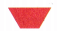do you need to make a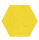?_________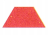make a.
Explanation:Two trapezoids need to join to make one hexagon.

Share and Show

Use pattern blocks. Draw to show the blocks. Write how many blocks you used.

Question 1.
How many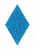make a?
_____3__make a.
Answer: Let’s use pattern blocks to visualize the situation and say that a hexagon is 1 whole. Since 3 rhombuses make a hexagon, 1 rhombus represents and 2 rhombuses represent. For example, because the rhombus is one-third of a hexagon, we can think of the fraction “one-third” as “one rhombus.” For a hexagon, “third” and “rhombus” are two names for the same thing.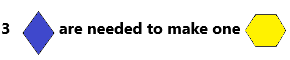Question 2.
How manymake a?
__________make a.
Answer: A trapezoid contains three triangles, or more depending on how you divide it. Where its longer parallel side is at the bottom, two triangles point up and one points down.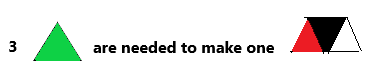Problem Solving

Use pattern blocks. Draw to show the blocks. Write how many blocks you used.

Question 3.
How manymake a?
____2______make a.
Answer: Yes, you will need 2 triangles for every hexagon.we can break this image into six small triangles each formed by one of the sides of the hexagon and each of the triangles is divided in half by a line. we will count the number of triangles formed by each part and by taking two or more such parts together.

Question 4.
How manymake a?
__________make a.
Answer: Two equilateral triangles can form a rhombus- it can also be formed by using a higher number of isosceles triangles.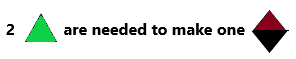Question 5.
H.O.T. Use me two times to make this shape. Which block am I? Circle a block to show your answer.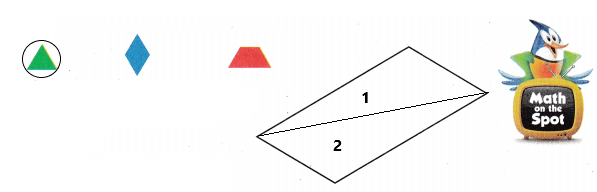Question 6.
H.O.T. Multi-Step Use these pattern blocks to make the shape. Write how many times you used each block.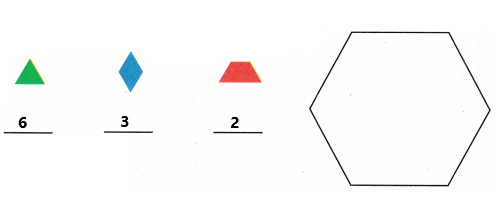Use pattern blocks. Choose the correct answer.

Question 7.
Use someto make a larger triangle. Draw the shape you made. Then draw a triangle with a different shape.
Answer: Triangles are just shaping with 3 (straight) sides. They can be big or small and can look somewhat different. Depending on the angles and the sides we can sort the triangles into different types.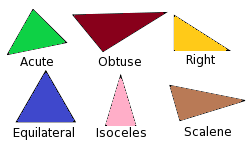Question 8.
Multi-Step Taylor used 3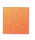to make a rectangle. Draw the shape he made. Then he used 4to make a square. Draw the shape he made.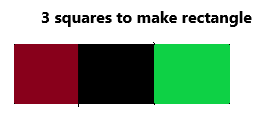Then he used 4 squares. Now it looks like in the below diagram.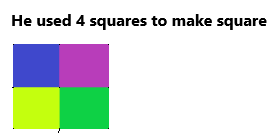Question 9.
Texas Test Prep How manydo you use to make a?
(A) 6
(B) 3
(C) 2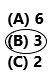Explanation:
Let’s use pattern blocks to visualize the situation and say that a hexagon is 1 whole. Since 3 rhombuses make a hexagon, 1 rhombus represents and 2 rhombuses represent.

TAKE HOME ACTIVITY • Have your child explain how he or she solved Exercises 1 and 2.
Answer: Make your child do the activity by giving things that they can do in two-dimensional shapes. And make them solve the problems given in the exercises.

### Texas Go Math Grade 1 Lesson 14.3 Homework and Practice Answer Key

Draw lines to show the shapes.

Question 1.
Draw lines to make this shape into 4.Answer: By dividing the square into 4 parts. we can make 4 squares.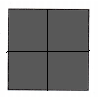Question 2.
Draw a line to make this shape into 2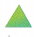.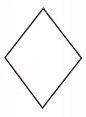Answer: Divide rhombus into two parts, then two triangles we can make easily.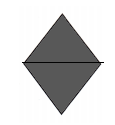Problem Solving

Question 3.
You could use me six times to make this shape. Which block am I? Circle a block to show your answer.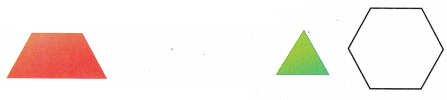Answer: Triangle. We use triangles 6 times to get a hexagon.Question 4.
You could use me three times to make this shape. Which block am I? Circle a block to show your answer.Answer: Three identical isosceles triangles are joined together to make a trapezium. Each triangle has a base of b cm and a height of h cm.Lesson Check

Question 5.
Jasmine makes this figure. Which shapes did she use?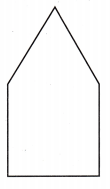(A) triangle and square
(B) rhombus and rectangle
(C) hexagon and rectangleQuestion 6.
How many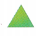make a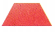?
(A) 2
(B) 4
(C) 3